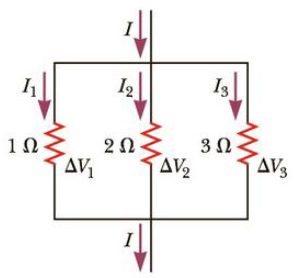Chapter 18, Problem 5CQ

Chapter
Section
Textbook Problem

Electric current I enters a node with three resistors connected in parallel (Fig. CQ18.5). Which one of the following is correct? (a) I1 = I and I2 = I3 = 0. (b) I2 > I1 and I2 > I3. (c) ΔV1 < ΔV2 < ΔV3 (d) I1 > I2 > I3 > 0.Figure CQ18.5

To determine
The role of resistance in transmitting power over long distances.

Explanation

All the three resistances are connected in parallel combination. Hence, the potential difference across each of them is the same.

Conclusion:

From Ohm’s law, the current is inversely related to the resistance, since the potential difference is constant. Hence, current through the 1Ω resistance is the largest and the current through the 3Ω resistance is the smallest. Therefore, option (d) is correct.

In a parallel circuit, the total current is not equal to the current through an individual resistance

Still sussing out bartleby?

Check out a sample textbook solution.

See a sample solution

The Solution to Your Study Problems

Bartleby provides explanations to thousands of textbook problems written by our experts, many with advanced degrees!

Get Started

Find more solutions based on key concepts